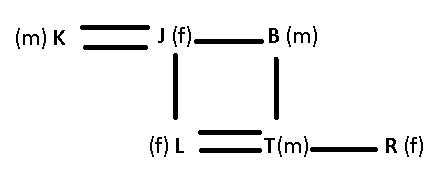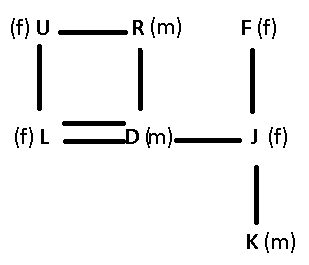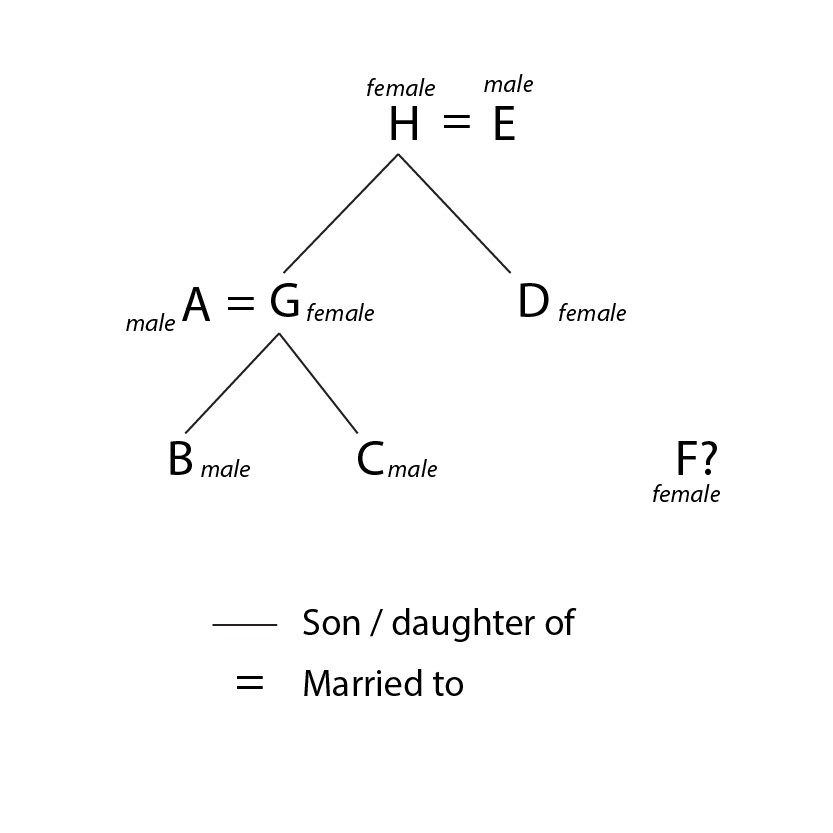# Blood Relations Questions for IBPS Clerk Set-3 PDF

0
621

## Blood Relations Questions for IBPS Clerk Set-3 PDF

Download important Blood Relations Questions PDF based on previously asked questions in IBPS Clerk and other Banking Exams. Practice Blood Relations Question and Answers for IBPS Clerk Exam.

Take Free IBPS Clerk Mock Test

Go to Free Banking Study Material (15,000 Solved Questions)

Instructions

Study the following information carefully and answer the questions given below :
K is the brother of J. J has only one daughter. J is the mother of L. L is the sister of T. B is the father of T. T is married to R.

Question 1: How is T related to K ?

a) Niece

b) Cannot be determined

c) Nephew

d) Son

e) Daughter

Question 2: How is J related to R ?

a) Cannot be determined

b) Sister-in-law

c) Mother

d) Aunt

e) Mother-in-law

Instructions

Study the following information carefully and answer the questions
given below :

R is married to U. U is the mother of L. L is the sister of D. U has only one daughter. D is married to J. K is the son of J. F is the mother of J.

Question 3: How is R related to K ?

a) Cannot be determined

b) Grandfather

c) Grandmother

d) Father

e) Uncle

Question 4: How is D related to F ?

a) Cannot be determined

b) Daughter

c) Daughter-in-law

d) Son-in-law

e) Son

Question 5: How is Ram’s mother’s niece’s father related to Ram?

a) Son

b) nephew

c) Cousin

d) Uncle.

e) Grandson

Question 6: Pointing towards a senior citizen, Ravi said, “This is my niece’s paternal grandmother’s sister-in-law.” How is Ravi related to the senior citizen?

a) brother

b) nephew

c) grandson

d) son-in-law

e) None of the above

Instructions

If A+B means “A is the husband of B”
A-B means “A is the sister of B”
A*B means “A is the brother of B”
A$B means “A is the father of B” Question 7: If A+B-C*D is true, how is A related to D? a) Cousin b) Father c) Brother d) Brother-in-law e) none of these Instructions Study the information carefully and answer the questions that follow: Lavkush is the husband of Devi. Lavkush has 3 children. Navin is the brother of Chang and Praneet. Praneet is the daughter of Lavkush. Question 8: How is Devi related to Chang? a) Sister b) Daughter c) Granddaughter d) Mother e) Can’t be determined Question 9: ‘P$\div$Q’ means ‘P is the brother of Q’, ‘P$\times$Q’ means ‘P is sister of Q’, ‘P – R’ means ‘P is the father of Q’ and ‘P + Q’ means ‘P is mother of Q’. Which of the following means S is maternal uncle of M ? a) M + R$\div$S b) S$\div$R + M c) S$\div$R$\times$M d) S + R – M e) None of these Instructions In a family of 8 people, G is the mother of B. H has two daughters – G and D. F is H’s only granddaughter. E is D’s father. C is the brother of B. B is A’s son. Question 10: How is D related to C? a) Uncle b) Aunt c) Brother d) Sister e) Can’t be determined Question 11: How many children does D have? a) 0 b) 1 c) 2 d) Either 0 or 1 e) Either 1 or 2 Question 12: If A+B means ‘A is the sister of B’,$A\times$B means ‘A is the wife of B’, A$\div$B means ‘A is the father of B’ and A-B means ‘A is the brother of B’, then which of the following expresses the relationship that ‘T is the daughter of P’ ? a)$P\times$Q$\div$R+S-T b)$P\times$Q$\div$R-T+S c)$P\times$Q$\div $R+T-S d)$P\times$Q$\div $R+S+T e) None of these Instructions (a) ‘M$\times$N’ means ‘N is brother of M’ (b) ‘M$\div$N’ means ‘M is father of N’. (c) ‘M – N’ means ‘M is sister of N’ (d) ‘M + N’ means ‘M is mother of N’ Question 13: Which of the following statements is/are superfluous to answer the previous question ” Is J the nephew of R” ? a) Only (a) b) Either (b) or (c) c) Both (b) and (d) d) Either (a) and (c) OR (b) and (d) e) Cannot be determined Question 14: Which of the following means J is nephew of R ? a) J$\times$T + R b) K$\times$R – J c) J$\times$T + R$\div$K d) J X T – R e) None of these Question 15: Pointing to Mukesh, Seema says ‘’He is the only grandchild of my father’’. How is Seema related to Mukesh ? a) Mother b) Aunt c) Grandmother d) Data inadequate e) None of these Answers & Solutions: 1) Answer (C) J has only one daughter and is mother of L, who is sister of T, => T is brother of L(female). => T is son of B(male) and husband of R. The flow chart will be : where, (m) represents male (f) represents female — represents married couple = represents siblings | represents childrenT is the son of K’s sister, => T is the nephew of K. Ans – (C) 2) Answer (E) J has only one daughter and is mother of L, who is sister of T, => T is brother of L(female). => T is son of B(male) and husband of R. The flow chart will be : where, (m) represents male (f) represents female — represents married couple = represents siblings | represents childrenJ is the mother of R’s husband, => J is mother-in-law of R. Ans – (E) 3) Answer (B) R is married to U, who is mother of L, => R is husband of U. U has only one daughter and L is the sister of D, => D is brother of L. D is married to J and has a son K. The flow chart will be : where, (m) represents male (f) represents female — represents married couple = represents siblings | represents childrenR is father of D, who is father of K, => R is grandfather of K. Ans – (B) 4) Answer (D) R is married to U, who is mother of L, => R is husband of U. U has only one daughter and L is the sister of D, => D is brother of L. D is married to J and has a son K. The flow chart will be : where, (m) represents male (f) represents female — represents married couple = represents siblings | represents childrenD is the husband of F’s daughter, => D is son-in-law of F. Ans – (D) 5) Answer (D) Ram’s mother’s niece is Ram’s cousin. Her father is Ram’s uncle. 6) Answer (E) Ravi’s niece’s paternal grandmother is Ravi’s sister-in-law’s mother. Her sister-in-law is Ravi’s sister-in-law’s aunt. So, Ravi is the nephew-in-law of the senior citizen. 7) Answer (D) A+B-C*D ==> A is the husband of B, B is the sister of C, C is the brother of D. ==>A is the brother-in-law of D. So correct option to choose is D. 8) Answer (D) Praneet is the daughter of Lavkush. Chang, Praneet and Navin are siblings. So, Chang, Naveen and Praneet are the children of Lavkush and Devi. So, Devi is Sam’s mother. 9) Answer (B) S$\div$R + M means ‘S is the brother of R’ and R is the mother of M. So, S is the maternal uncle of M. 10) Answer (B) From the information given, we can draw a family tree as shown below.Hence, D is C’s Aunt. 11) Answer (D) From the information given, we can draw a family tree as shown below.We don’t know if F is G’s or D’s daughter. Hence, D has either 0 or 1 child. 12) Answer (B)$P\times$Q$\div$R-T+S means P is the wife of Q and Q is father of R and R is the brother of T and T is the sister of S. So, P is the mom and Q is the dad of R, T and S. Hence (b) is the correct answer. 13) Answer (E) As we have seen in the previous question, this question cannot be answered even after using all the statements. Hence, option E is the right answer. 14) Answer (E) Let us start with the first option. Option A: J X T + R means that J is the brother of T who is the mother of R. The gender of R cannot be determined ( R can be nephew/niece). Option B: K X R – J means that K is the brother of R who is the sister of J. All three of them are siblings. Option C: J X T + R$\div\$ K means that J is the brother of T. T is the mother of R. R is the father of K. Hence, J is the uncle of R. But the question asks whether J is the nephew of R.

Option D: J X T – R means that J is the brother of R. hence, the required relationship is not obtained.

Therefore, the correct answer is option E – none of the above.